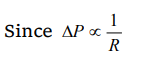## Mechanical Properties of Fluids Questions and Answers Part-11

1. If the surface of a liquid is plane, then the angle of contact of the liquid with the walls of container is
a) Acute angle
b) Obtuse angle
c) 90°
d) 0°

Explanation: Tangent drawn at point of contact makes 0° with wall of container

2. A soap bubble assumes a spherical surface. Which of the following statement is wrong
a) The soap film consists of two surface layers of molecules back to back
b) The bubble encloses air inside it
c) The pressure of air inside the bubble is less than the atmospheric pressure; that is why the atmospheric pressure has compressed it equally from all sides to give it a spherical shape
d) Because of the elastic property of the film, it will tend to shrink to as small a surface area as possible for the volume it has enclosed

Explanation: The pressure of air inside the bubble is less than the atmospheric pressure; that is why the atmospheric pressure has compressed it equally from all sides to give it a spherical shape

3. If two soap bubbles of different radii are in communication with each other
a) Air flows from larger bubble into the smaller one
b) The size of the bubbles remains the same
c) Air flows from the smaller bubble into the large one and the larger bubble grows at the expense of the smaller one
d) The air flows from the larger

Explanation:4. The surface tension of soap solution is $25\times10^{-3}Nm^{-1}$  . The excess pressure inside a soap bubble of diameter 1 cm is
a) 10 Pa
b) 20 Pa
c) 5 Pa
d) None of the above

Explanation:5.When two soap bubbles of radius $r_{1}$ and $r_{2}$ $\left(r_{2}>r_{1}\right)$  coalesce, the radius of curvature of common surface is
a) $r_{2}-r_{1}$
b) $\frac{r_{2}-r_{1}}{r_{1}r_{2}}$
c) $\frac{r_{1}r_{2}}{r_{2}-r_{1}}$
d) $r_{2}+r_{1}$

Explanation: $\frac{r_{1}r_{2}}{r_{2}-r_{1}}$

6. The excess pressure due to surface tension in a spherical liquid drop of radius r is directly proportional to
a) r
b) $r^{2}$
c) $r^{-1}$
d) $r^{-2}$

Explanation: $r^{-1}$

7. A long cylindrical glass vessel has a small hole of radius 'r' at its bottom. The depth to which the vessel can be lowered vertically in the deep water bath (surface tension T) without any water entering inside is
a) $4T/ \rho rg$
b) $3T/ \rho rg$
c) $2T/ \rho rg$
d) $T/ \rho rg$

Explanation:8. If the surface tension of a soap solution is 0.03 MKS units, then the excess of pressure inside a soap bubble of diameter 6 mm over the atmospheric pressure will be
a) Less than $40 N/m^{2}$
b) Greater than $40 N/m^{2}$
c) Less than $20 N/m^{2}$
d) Greater than $20 N/m^{2}$

Explanation:9. The excess of pressure inside a soap bubble than that of the outer pressure is
a) $\frac{2T}{r}$
b) $\frac{4T}{r}$
c) $\frac{T}{2r}$
d) $\frac{T}{r}$

Explanation: $\frac{4T}{r}$